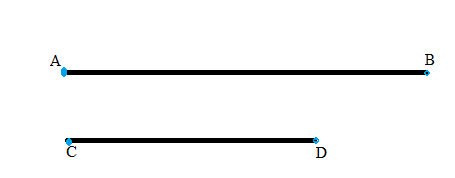Chapter A.3, Problem 1EElementary Geometry For College St...

7th Edition
Alexander + 2 others
ISBN: 9781337614085

Solutions

Chapter
SectionElementary Geometry For College St...

7th Edition
Alexander + 2 others
ISBN: 9781337614085
Textbook Problem

If line segment AB and line segment CD in the accompanying drawing are drawn to scale, what does intuition tell you about the length of these segments?To determine

To write:

The intuition about the following length of these segments,Explanation

Consider the following line segment AB and line segment CD,

The above line segment shows that the line segment AB is larger line than the line segment CD.

That is in symbolically AB

Still sussing out bartleby?

Check out a sample textbook solution.

See a sample solution

The Solution to Your Study Problems

Bartleby provides explanations to thousands of textbook problems written by our experts, many with advanced degrees!

Get Started

In Problems 7-20, find each limit, if it exists.

Mathematical Applications for the Management, Life, and Social Sciences

If f(x) = exg(x), where g(0) = 2 and g'(0) = 5, find f'(0).

Single Variable Calculus: Early Transcendentals, Volume I

Fill in each blank: 9000lb=tons

Elementary Technical Mathematics

Differentiate the function. f(t) = 2t3 3t2 4t

Single Variable Calculus: Early Transcendentals

Sometimes, Always, or Never: The antiderivative of an elementary function is elementary.

Study Guide for Stewart's Single Variable Calculus: Early Transcendentals, 8th

Define a construct and explain the role that constructs play in theories.

Research Methods for the Behavioral Sciences (MindTap Course List)